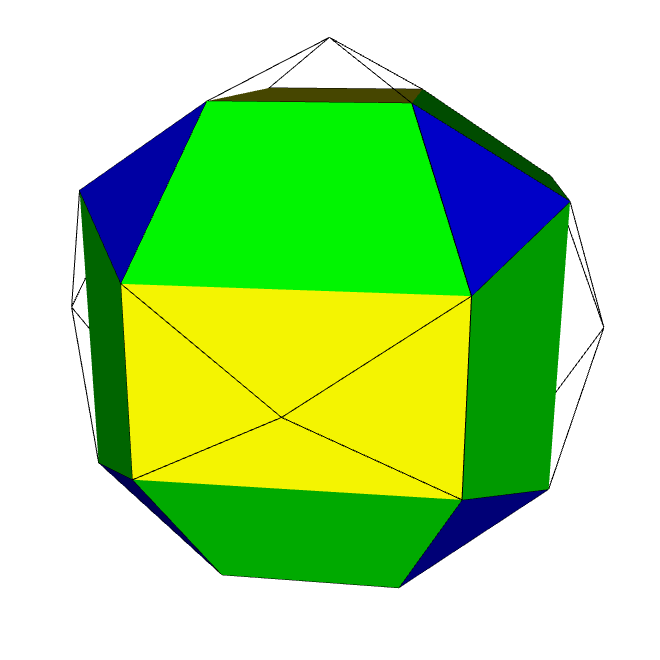Acronym pysnic Name pyritosnub cube,pyritohedral sirco variant,hexadiminished id Circumradius (1+sqrt(5))/2 = 1.618034Confer uniform variant: sirco   variations: xwX wXx Xxw&#zh   general polytopal classes: isogonal ExternallinksThis polyhedron is being obtained when the id gets diminished at the vertices of an inscribed oct. Here the edge ratios are x : x : f = 1 : 1 : (1+sqrt(5))/2. The uniform variant of this polyhedron however is the sirco (then with ratios 1 : 1 : 1). Note that a further polyhedron with this same combinatoric topology, but again different edge ratios, would occur as mere vertex alternation, i.e. as snubbing without subsequent resizement, when being applied to girco. That one then (xwX wXx Xxw&#zh) would use edge size ratios x : h : w = 1 : sqrt(3) : 1+sqrt(2) instead.

Incidence matrix according to Dynkin symbol

xfF fFx Fxf&#zx   → height = 0
F = x+f

o.. o.. o..       | 24 |  1  1  2 | 1  2 1
------------------+----+----------+-------
... f.. ...     & |  2 | 12  *  * | 1  1 0
... ... x..     & |  2 |  * 12  * | 1  1 0
oo. oo. oo.&#x  & |  2 |  *  * 24 | 0  1 1
------------------+----+----------+-------
... f.. x..     & |  4 |  2  2  0 | 6  * *
... ... xf.&#x  & |  4 |  1  1  2 | * 12 *
ooo ooo ooo&#x    |  3 |  0  0  3 | *  * 8